Quick Notes Sigma and Pi Bonding

• Sigma, σ, bonds are a type of covalent bond that form from the direct overlap of two atomic orbitals from two atoms.
•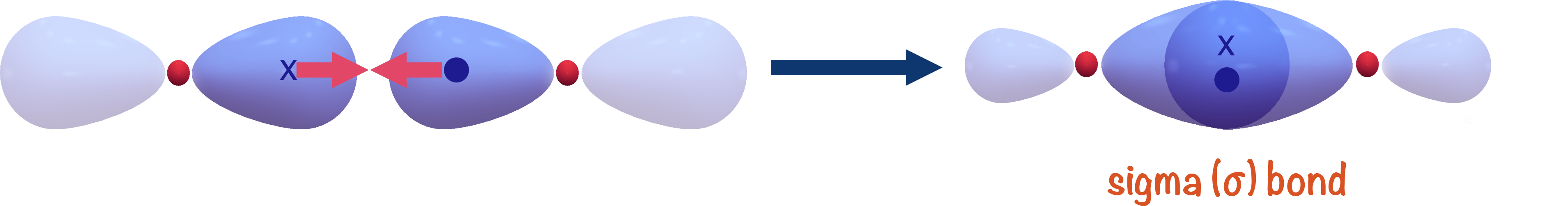• Pi, π , bonds are a type of covalent bond that form from the sideways overlap of two p-shaped orbitals from two atoms.
•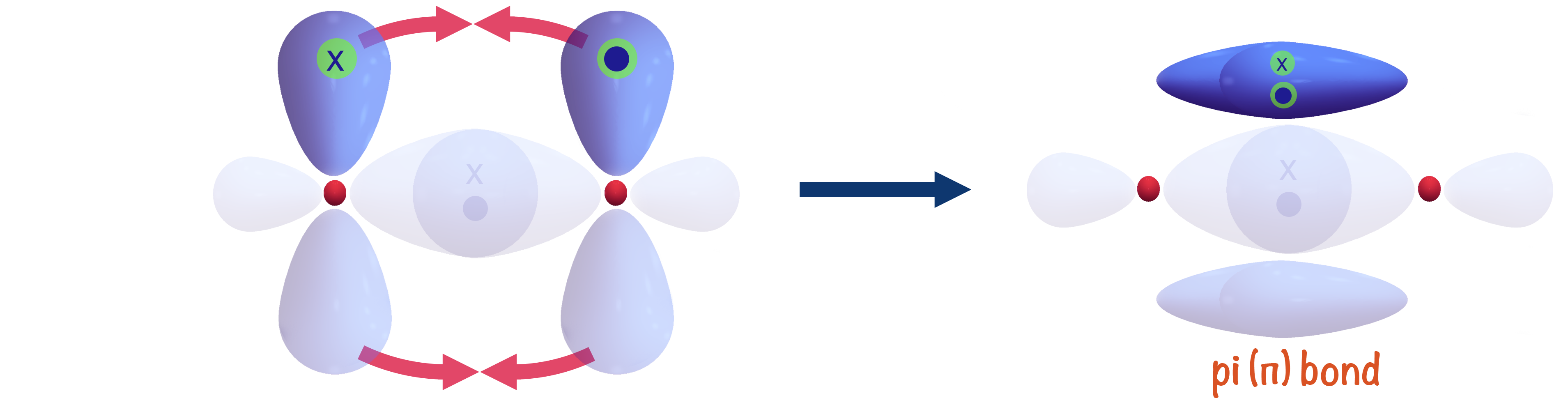• Two atoms that form a sigma and pi-bond between themselves will have a 'double bond' between them and will be harder to break apart than if they only had a sigma bond.
• Sigma bonds are stronger than pi-bonds.
• Shared electron pair in a sigma bond is closer to the positively charged nuclei of both atoms, meaning a stronger force of attraction between the electrons (negatively charged) and nuclei (positively charged).
• Shared electron pair in a pi-bond is further from the nuclei of both atoms, meaning a weaker force of attraction between the electrons and nuclei.
• Sigma bonds have free rotation whereas pi-bonds are unable to rotate (restricted rotation).
• High electron density in a pi-bond means it can be 'attacked' be electrophiles.

Full Notes Sigma and Pi Bonding

In order for a covalent bond to form, two atoms have to come close enough together to allow two atomic orbitals to 'overlap'.

There are two main ways orbitals can overlap when forming a bond: directly, leading to the forming of a sigma (σ) bond and sideways, leading to the forming of a pi (π) bond.

We will use the simple example of bonding in an oxygen molecule to show the forming of sigma and pi bonds. The carbon-carbon double bond is outlined at the bottom of the page.

## Sigma (σ) Bonds

Usually, when a 'single' covalent bond forms between two atoms, two atomic orbitals can simply directly overlap and one electron from each can end up in the new 'bonding orbital' that forms between the two atoms. This type of covalent bond is referred to as a 'sigma' bond, shown with the greek symbol σ.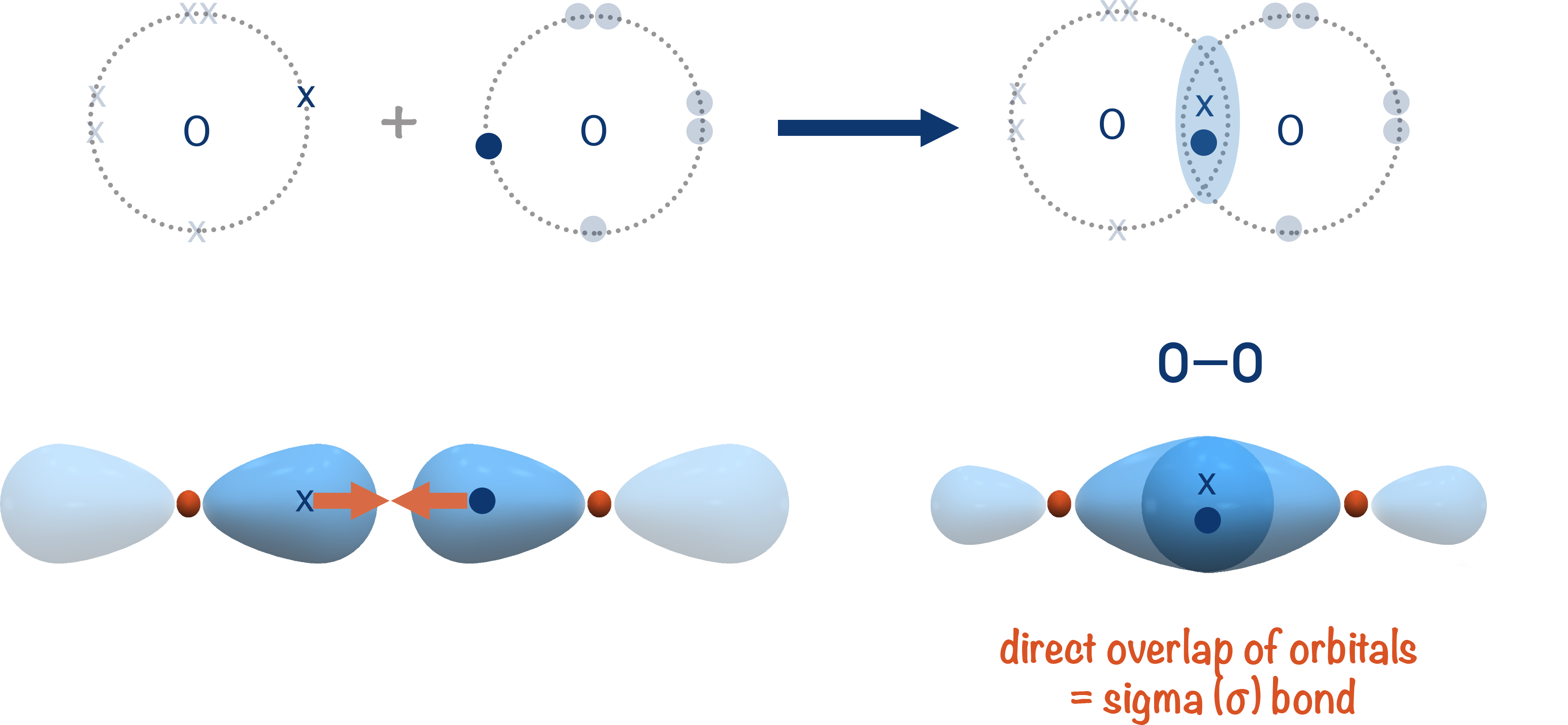The new bonding orbital that gets formed is close to the nucleus of each atom, giving maximum attraction between the positively charged nuclei and negatively charged electrons in the orbital.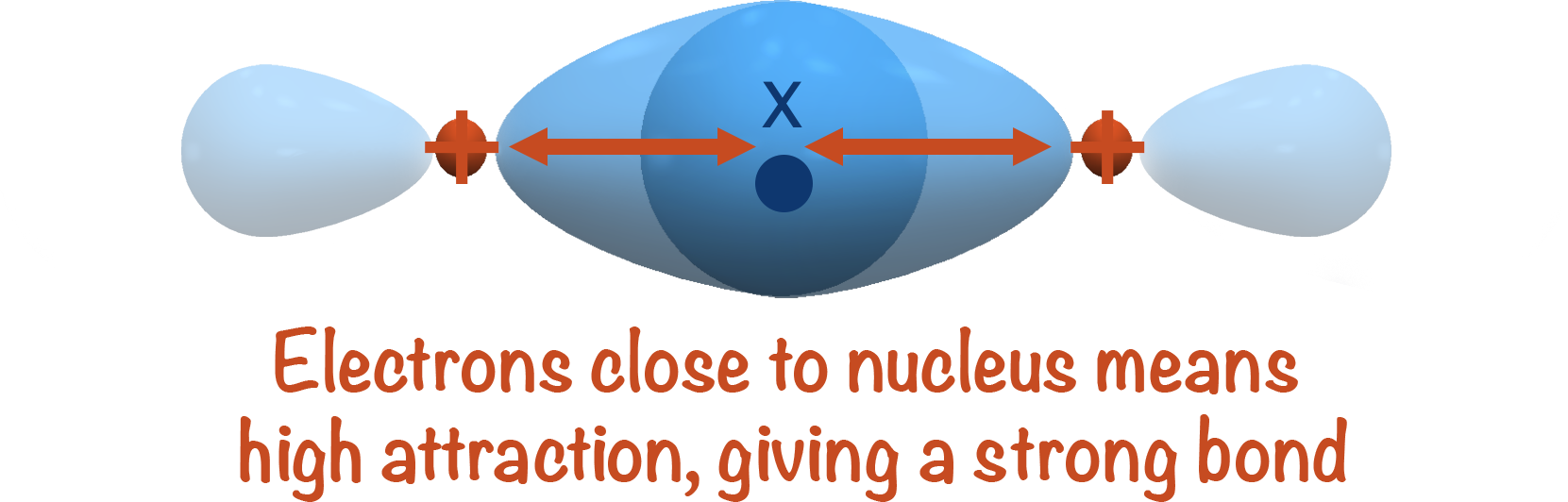This high level of attraction is hard to overcome, making sigma bonds strong and hard to break.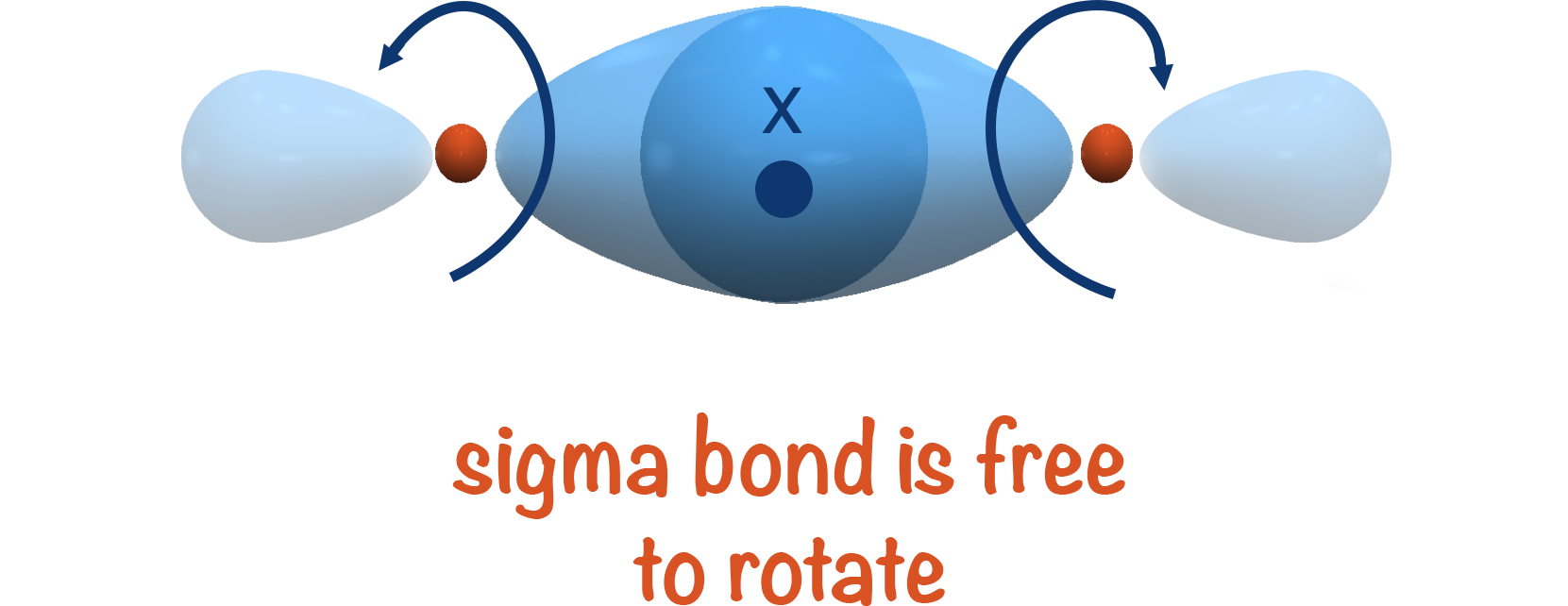As the shared electrons are in the space directly between the two atoms, each atom can be 'twisted' and the bond isn't affected. This means sigma bonds are free to rotate.

'Single' covalent bonds are sigma bonds as two bonding atoms will always try and arrange themselves to enable maximum overlap of their two orbitals when forming a bond.

## Pi (π) Bonds

Sometimes, two atoms can share more than one pair of electrons, forming double or even triple covalent bonds.

Remember, to form a covalent bond, two atomic orbitals from each atom have to 'overlap' and create a new, bonding orbital (space where the electron pair can exist between the two atoms).

Once a sigma bond has been formed between two atoms, any other bonds betweent them would likely have to be formed by the overlap of two p-shaped orbitals.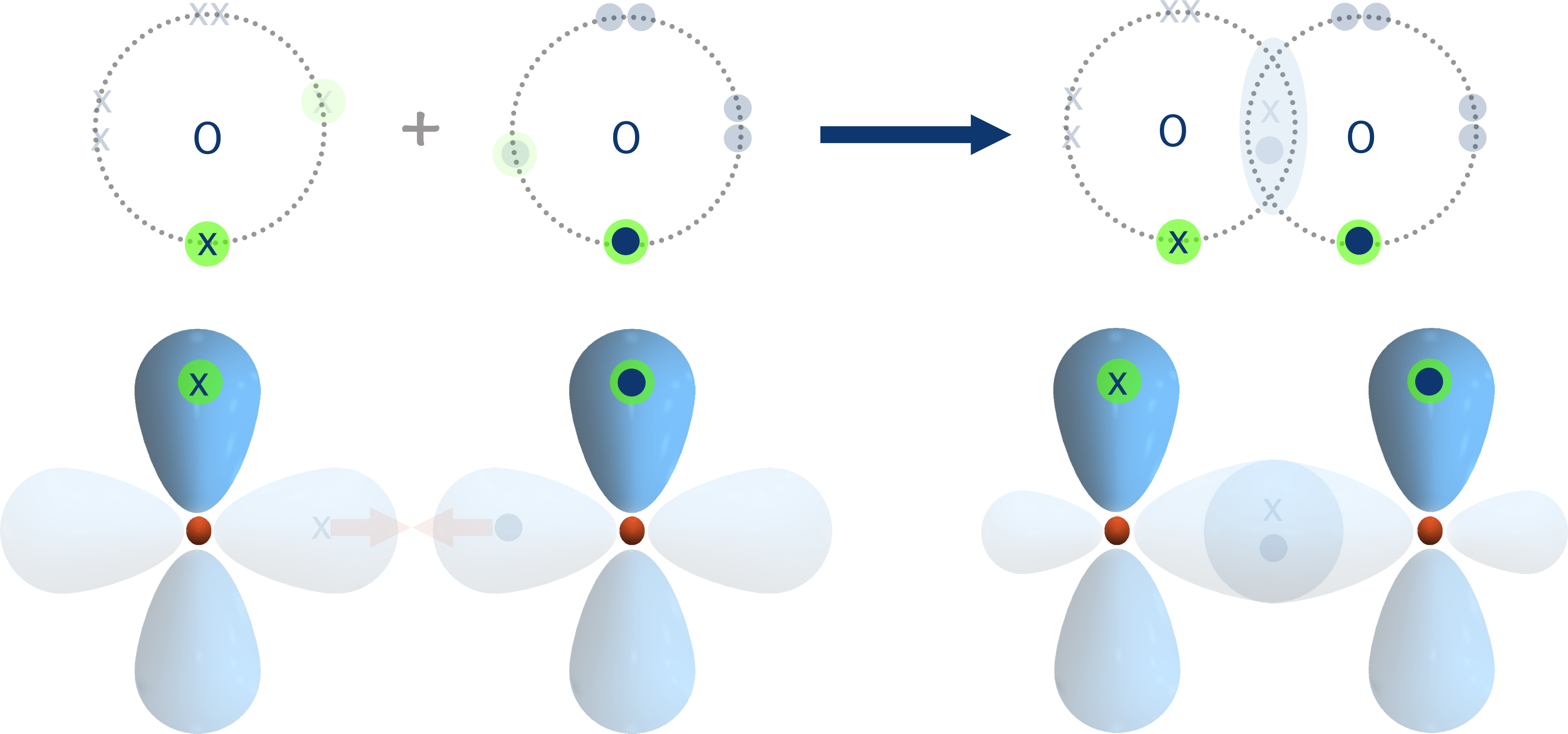Due to the arrangement and geometry of p-shaped orbitals around an atoms nucleus, if an orbital from each atom has already overlapped and formed a sigma (σ) bond, the remaining half filled p-orbital(s) must be at ninenty degrees to the sigma bond. This means the orbitals from each atom are unable to directly overlap as they arn't 'pointing' towards each other.

To overcome this, each p-shaped orbital has to 'bend inwards' slightly and the orbitals overlap sideways.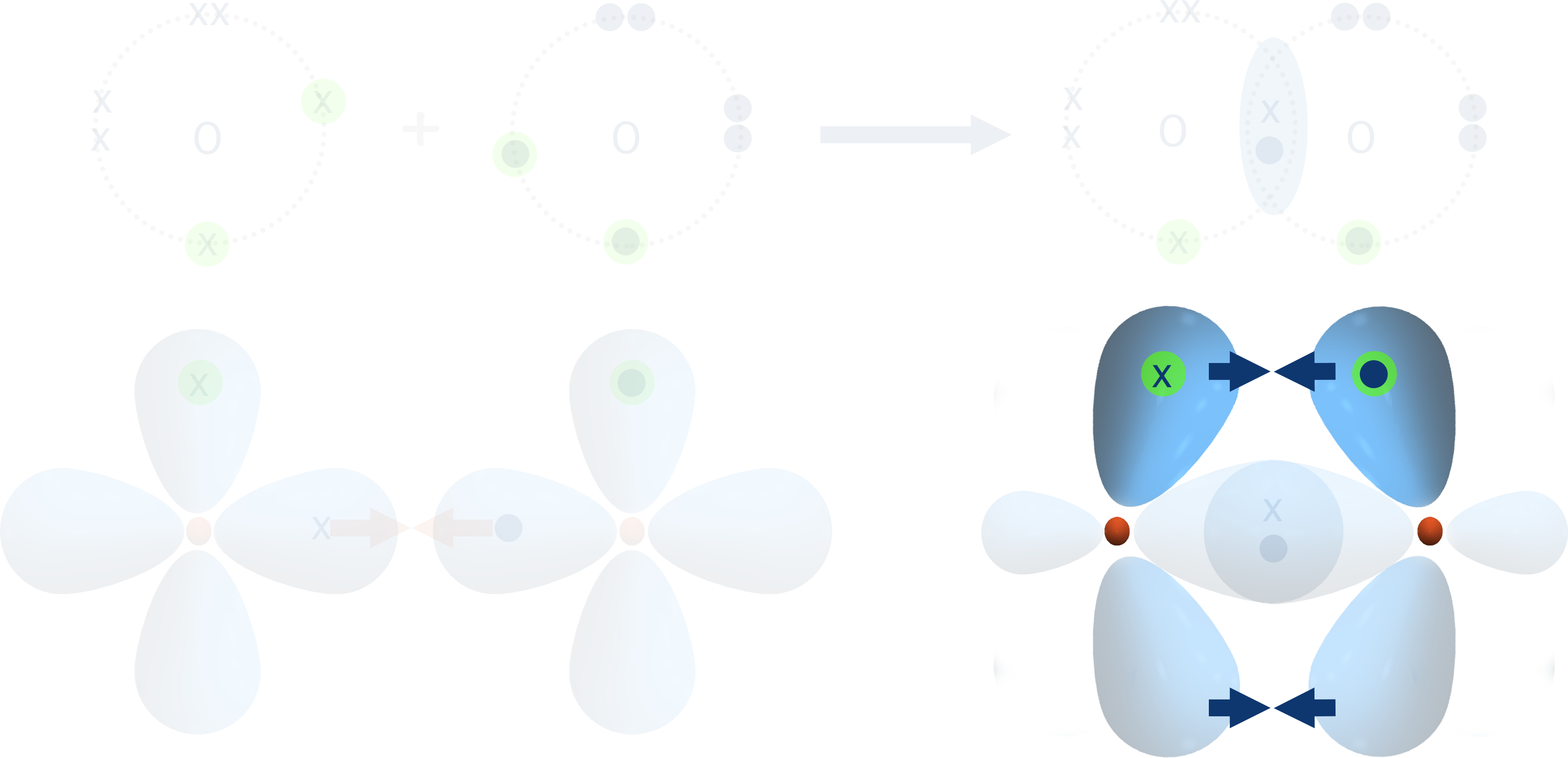The new bonding orbital formed exists in two regions - one above and one below the sigma bond already formed between the two atoms.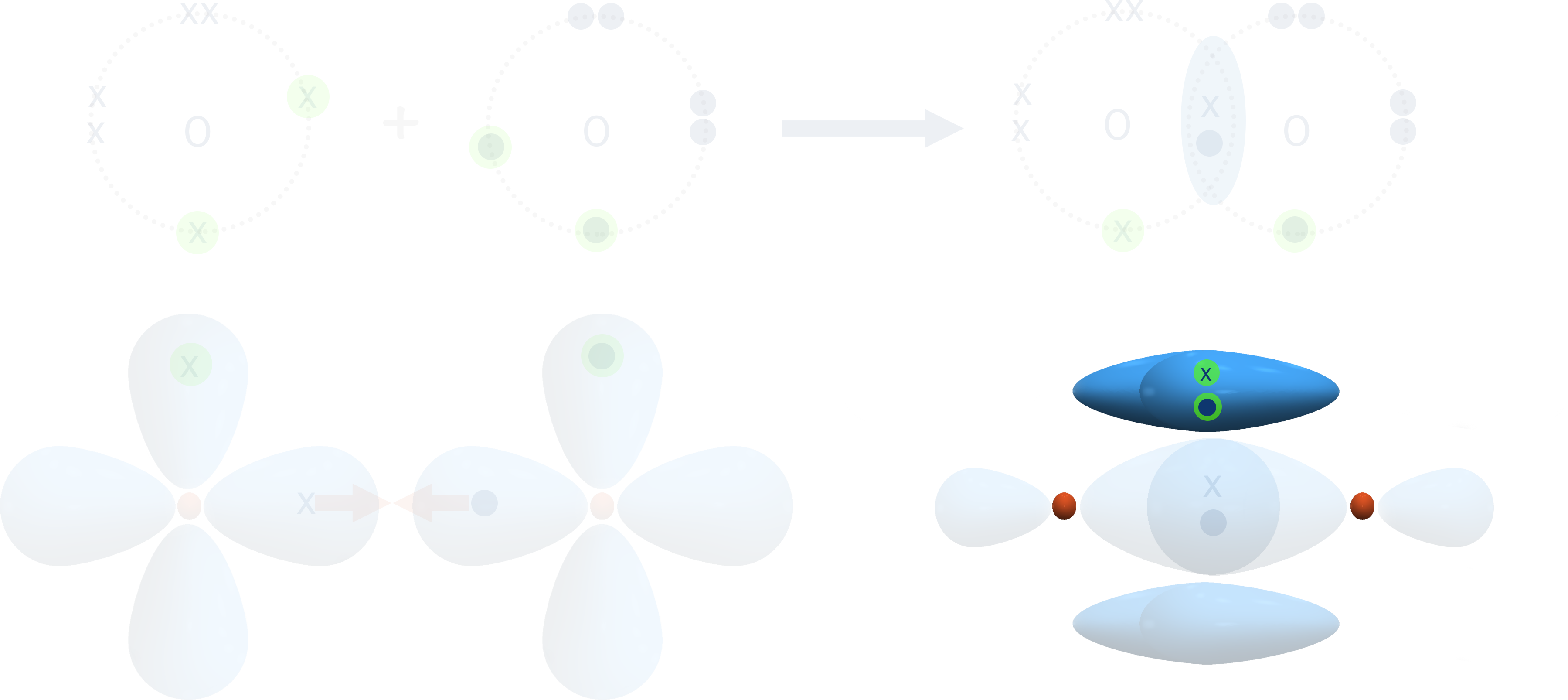This is referred to as a pi-bond, shown with the greek symbol π.

As the electron pair in a pi-bond is further from the nucleus of each atom, there is a weaker force of attraction between them and the positively charged nucleus compared to in the sigma bond. This makes the pi-bond weaker than a sigma bond and easier to break.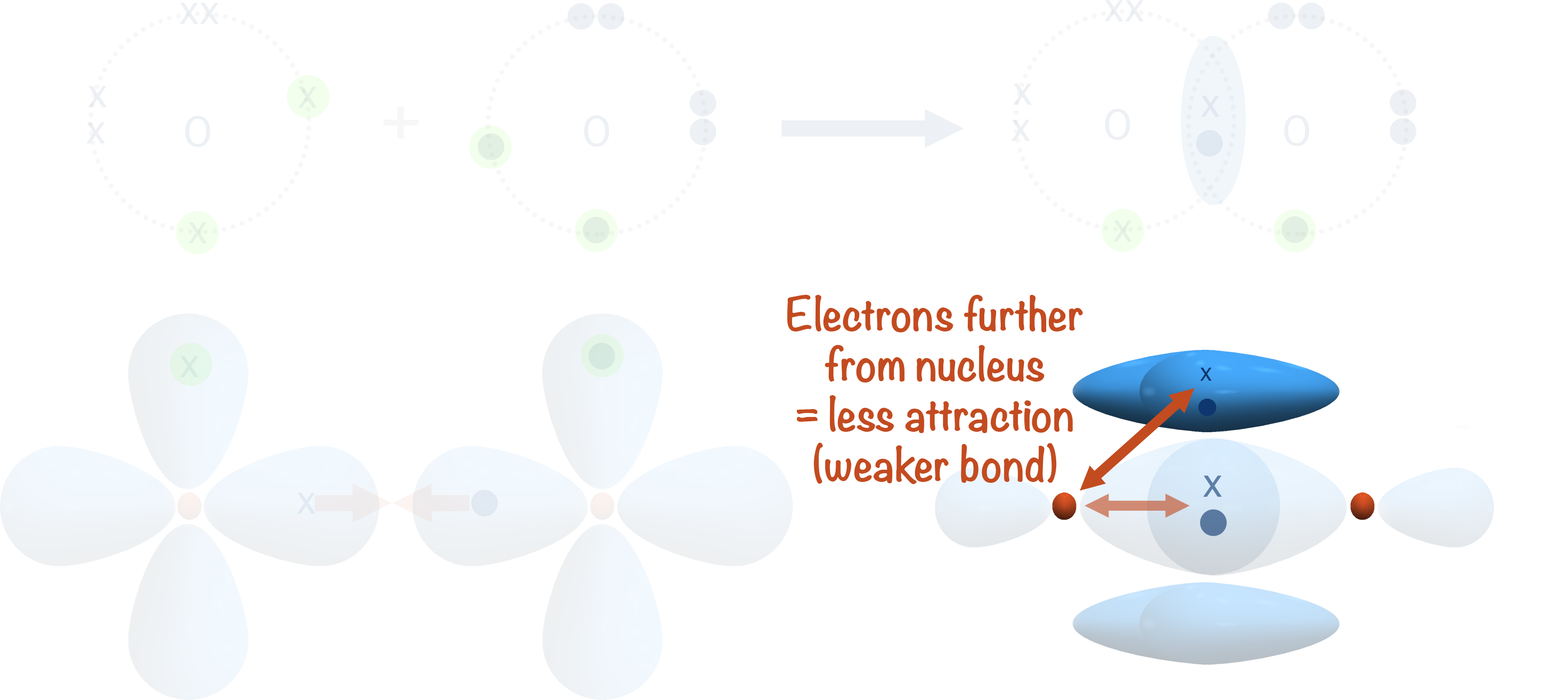As the pi-bond has two regions of electron density (one above and one below the plane of the sigma bond), it is impossible to rotate one of the atoms without breaking the pi-bond. This means pi-bonds have restricted rotation and are unable to rotate freely (unlike sigma bonds that are able to).

This restricted rotation of pi-bonds leads to stereoisomerism in certain compounds (see stereoisomerism).

## The Carbon-Carbon Double Bond

If two carbon atoms form both a sigma and pi-bond with each other, there is a 'double' bond between them. We show this with a double line. One of the lines repesents a sigma bond, the other a pi-bond.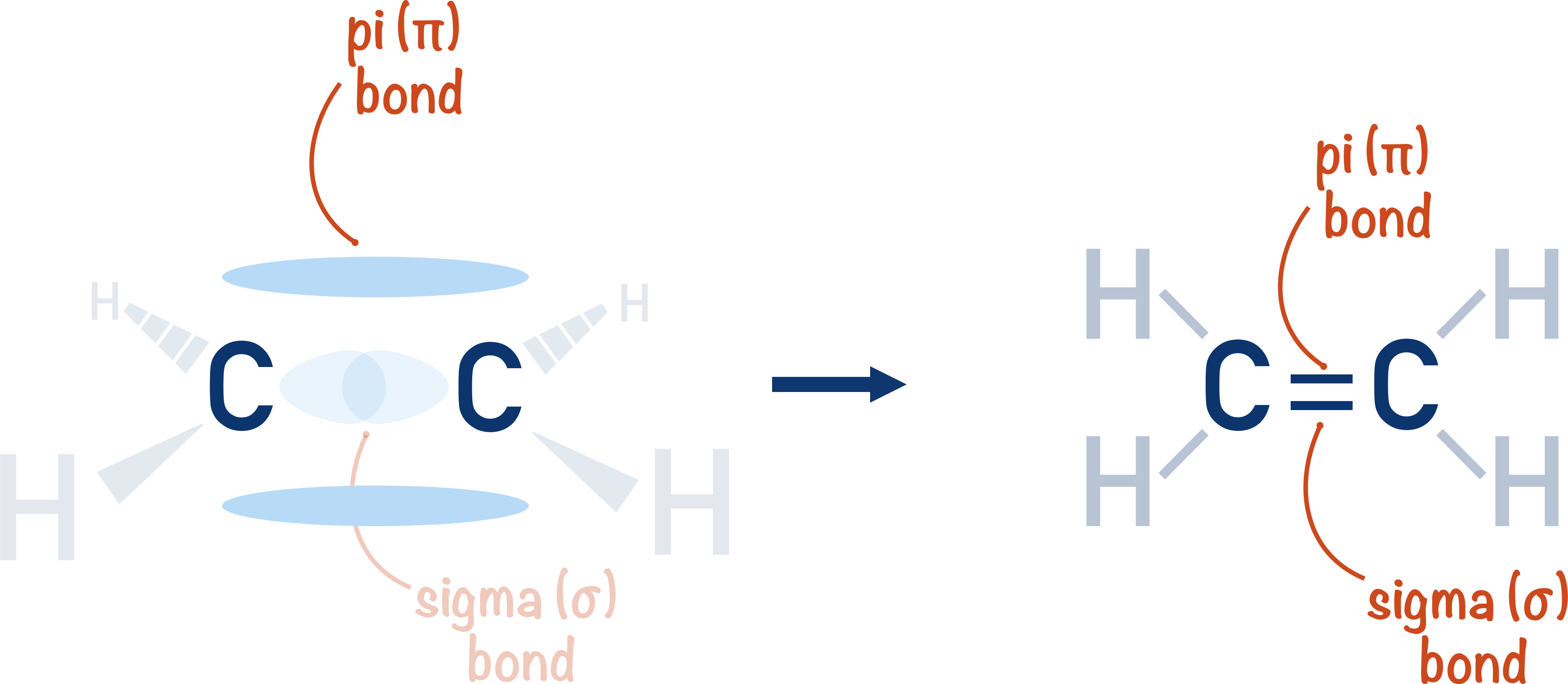Due to the presence of a pi-bond, the two atoms will be held together more tightly than when only singly bonded with a sigma bond. As the pi-bond is weaker than a sigma-bond however, the carbon-carbon double bond isn't twice as strong as a carbon-carbon single bond.

This means in a reaction the pi-bond is, relatively, easy to break and if it does break, a sigma bond is still left between the two carbon atoms.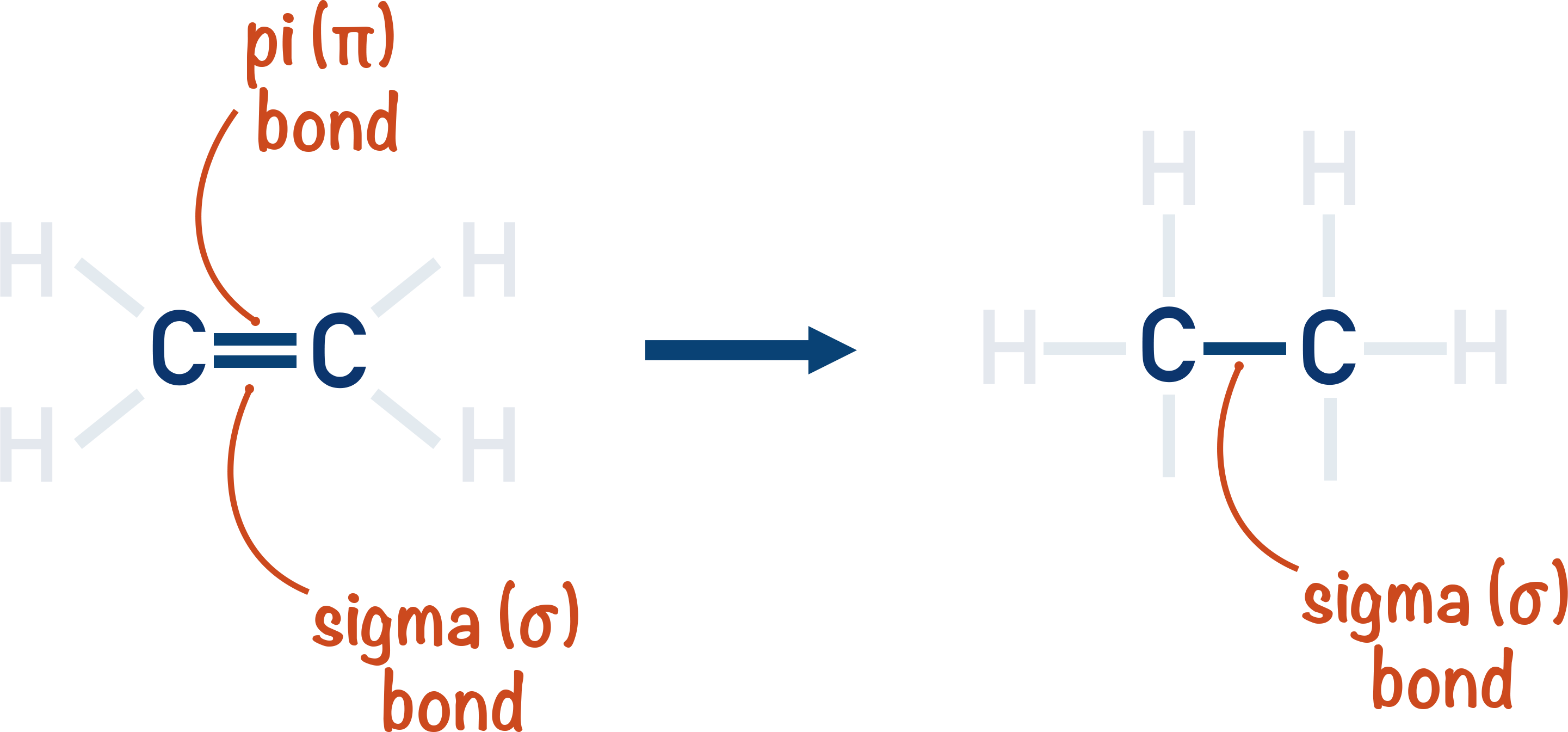This means hydrocarbons that contain a carbon-carbon double bond (alkenes) are more reactive than those that don't (alkanes).

The high electron density of pi-bond means carbon-carbon double bonds react with electrophiles ).